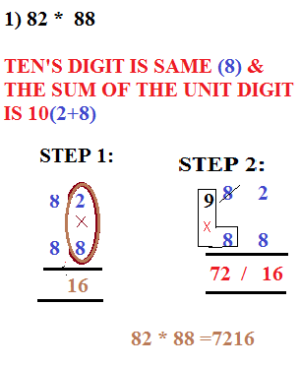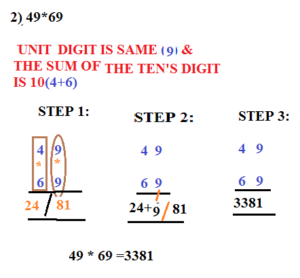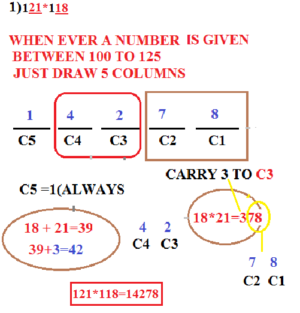# MULTIPLICATION TECHNIQUE:SPECIAL CASE – LEARN SERIES

Hi Bankersdaily Aspirants,

Learning is a step by step process and you should gradually progress in that to ace the process. As you all know we have started a new Learn Series to help with your preparations for various exams like IBPS PO , IBPS RRB Scale I officer , IBPS RRB Office Assistant , IBPS Clerk , IBPS SO , SBI PO , SBI Clerk , SBI SO and many other exams in this type. Nevertheless this series will also contribute you all the tips and tricks to every miscellaneous sections and also all the important topics which are asked in the exams. If you haven’t looked at the Learn series , Please Click the below link to learn the new concepts.

## INTRODUCTION:

Friends today we are going to look,Special Case in Multiplication Technique,Before that let us have a glance on Multiplication Technique which we had discussed in the Previous Article

SPECIAL CASES IN MULTIPLICATION TECHNIQUES

1.Multiplying  2 numbers Whose Ten’s Digit is Same and Sum of Unit Digit is equal to 10.

2.Multiplying 2 numbers Whose Sum of Ten’s Digit is 10 and Unit Digit is Same.

3.Multiplying 2 numbers between 100 to 125

### Multiplying Two Numbers Whose Ten’s Digit is Same and Sum of the Unit Digit is Equal to 10:## #.2 TECHNIQUE 2:

### MULTIPLYING 2 NUMBERS WHOSE UNIT IS SAME AND SUM OF TEN’S DIGIT IS 10:### MULTIPLYING 2 NUMBERS BETWEEN 100 – 125:I think these Techniques will be useful to you,Let Us see some other Special Cases in Next Article